Function Repository Resource:

# RandomWeightedGraph

Create a random weighted graph

Contributed by: Peter Burbery
 ResourceFunction["RandomWeightedGraph"][{v,e},randomfunction] generates a random graph with v vertices and e edges with edge weights given by randomfunction. ResourceFunction["RandomWeightedGraph"][graphdistribution,randomfunction] generates a random graph following graphdistribution. ResourceFunction["RandomWeightedGraph"][spec,randomfunction,k] generates k random graphs. ResourceFunction["RandomWeightedGraph"][spec,randomfunction,dims] generates an array with dimensions dims.

## Details

randomfunction should be based on RandomReal or RandomInteger, for example RandomReal[{1,7}]&.
The function accepts the same options as Graph.

## Examples

### Basic Examples (2)

Make a random graph:

 In:=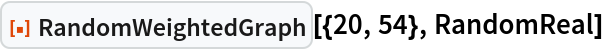Out=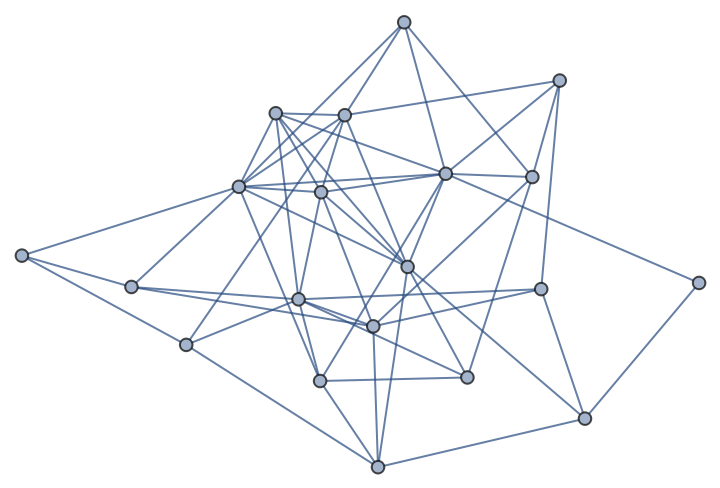Make a random graph with edge weights between 1 and 7:

 In:=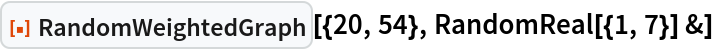Out=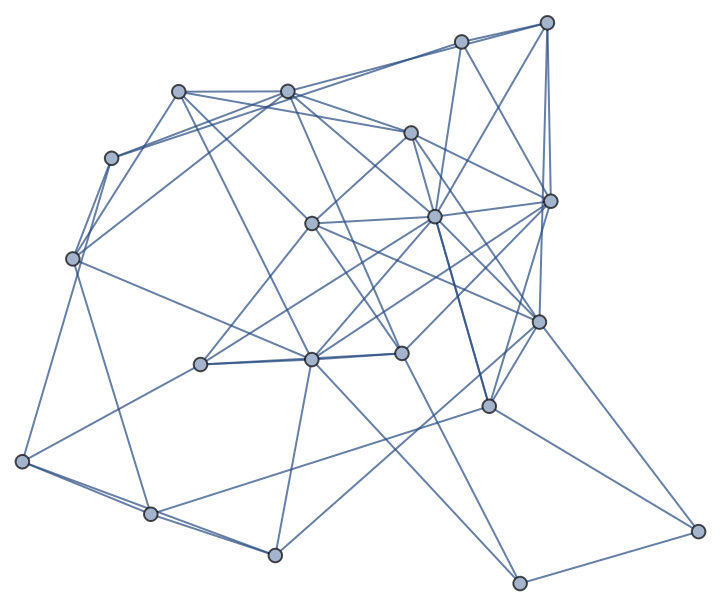### Scope (5)

Make a random graph with integer weights:

 In:=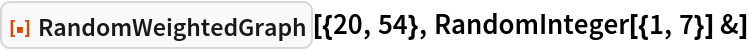Out=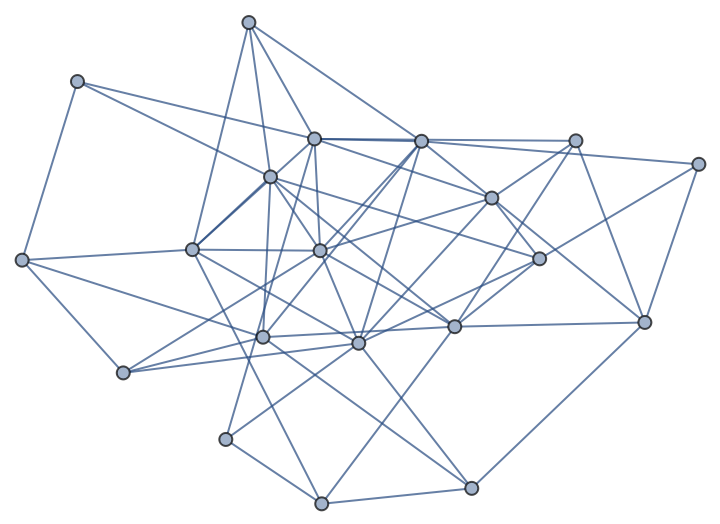Make a list of random graphs:

 In:=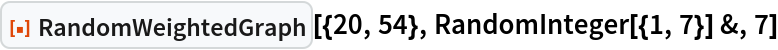Out=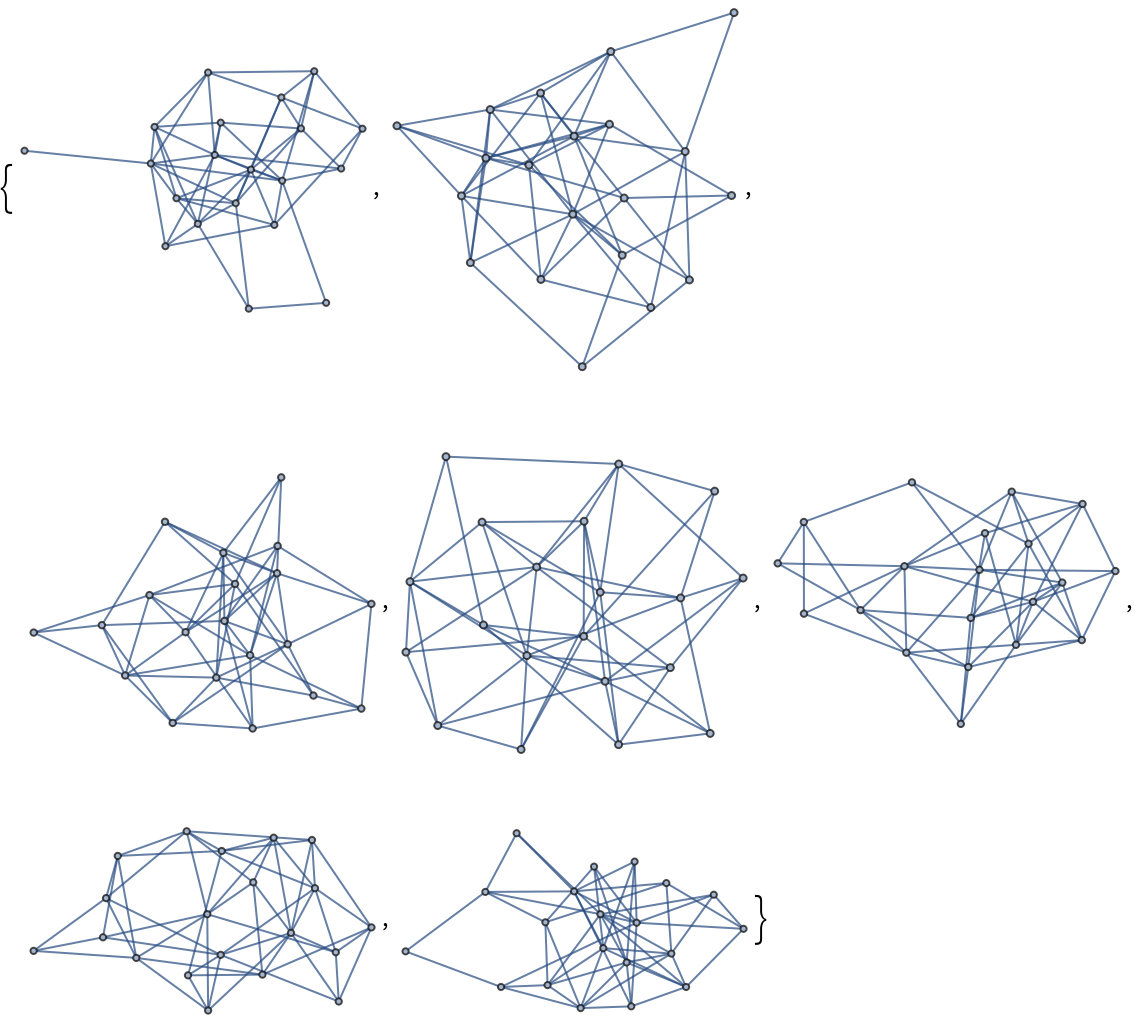Make an array of random graphs:

 In:=Out=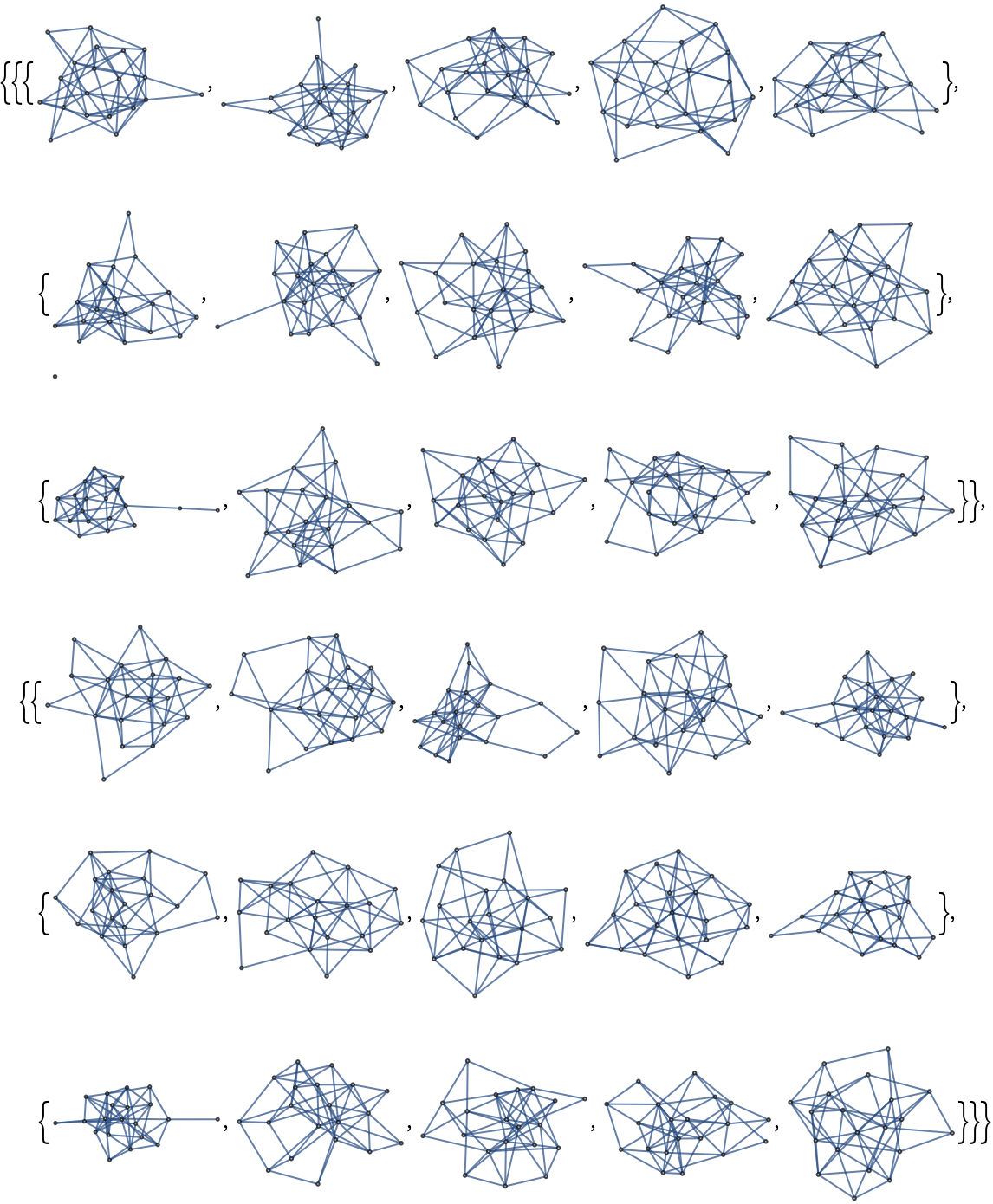Make a random graph with a graph distribution:

 In:=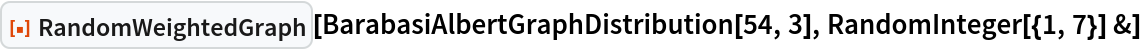Out=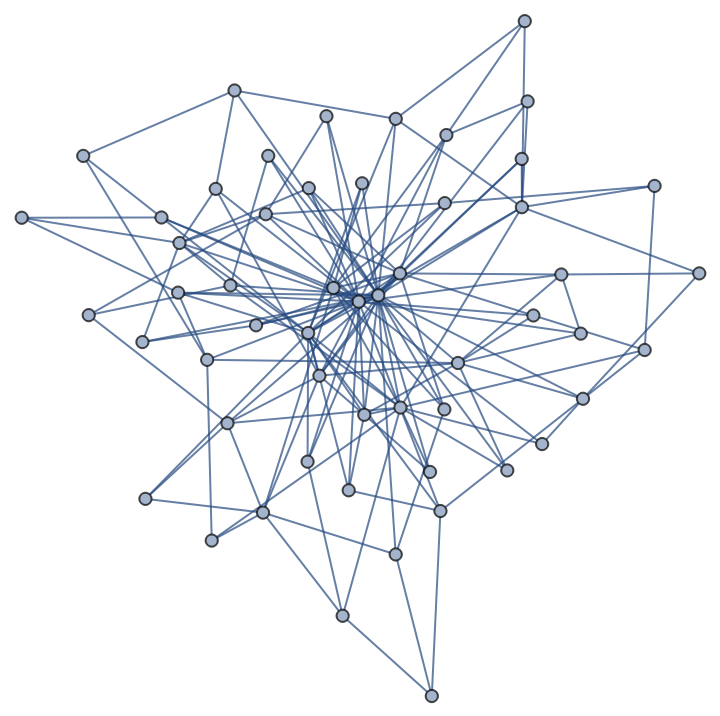Create a random weighted graph with integer weights with edge labels:

 In:=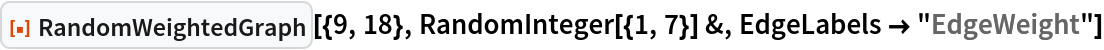Out=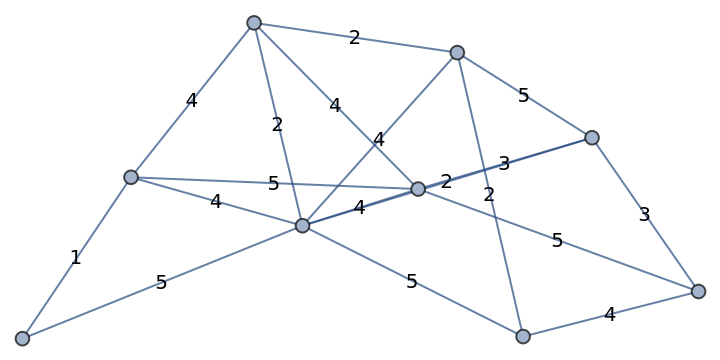### Properties and Relations (3)

Create a random weighted graph:

 In:=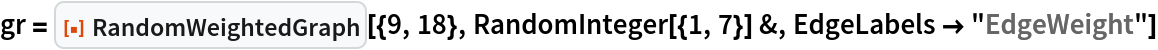Out=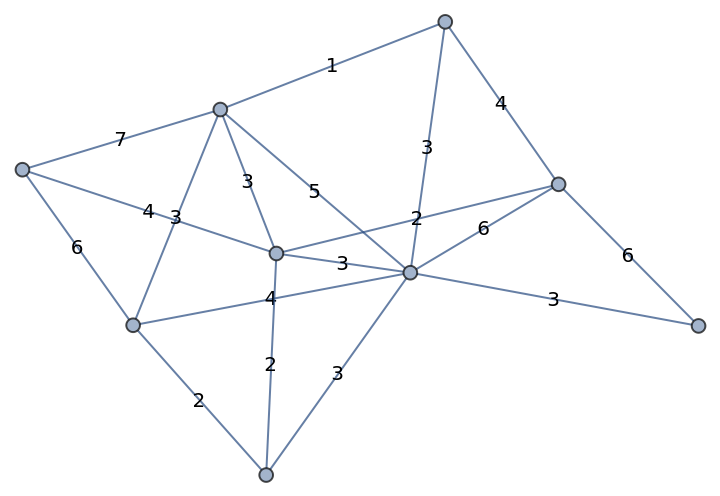Find a solution to the undirected Chinese Postman problem for this graph:

 In:=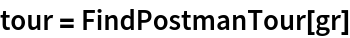Out=Make a table highlighting the steps by constructing directed graphs:

 In:=Out=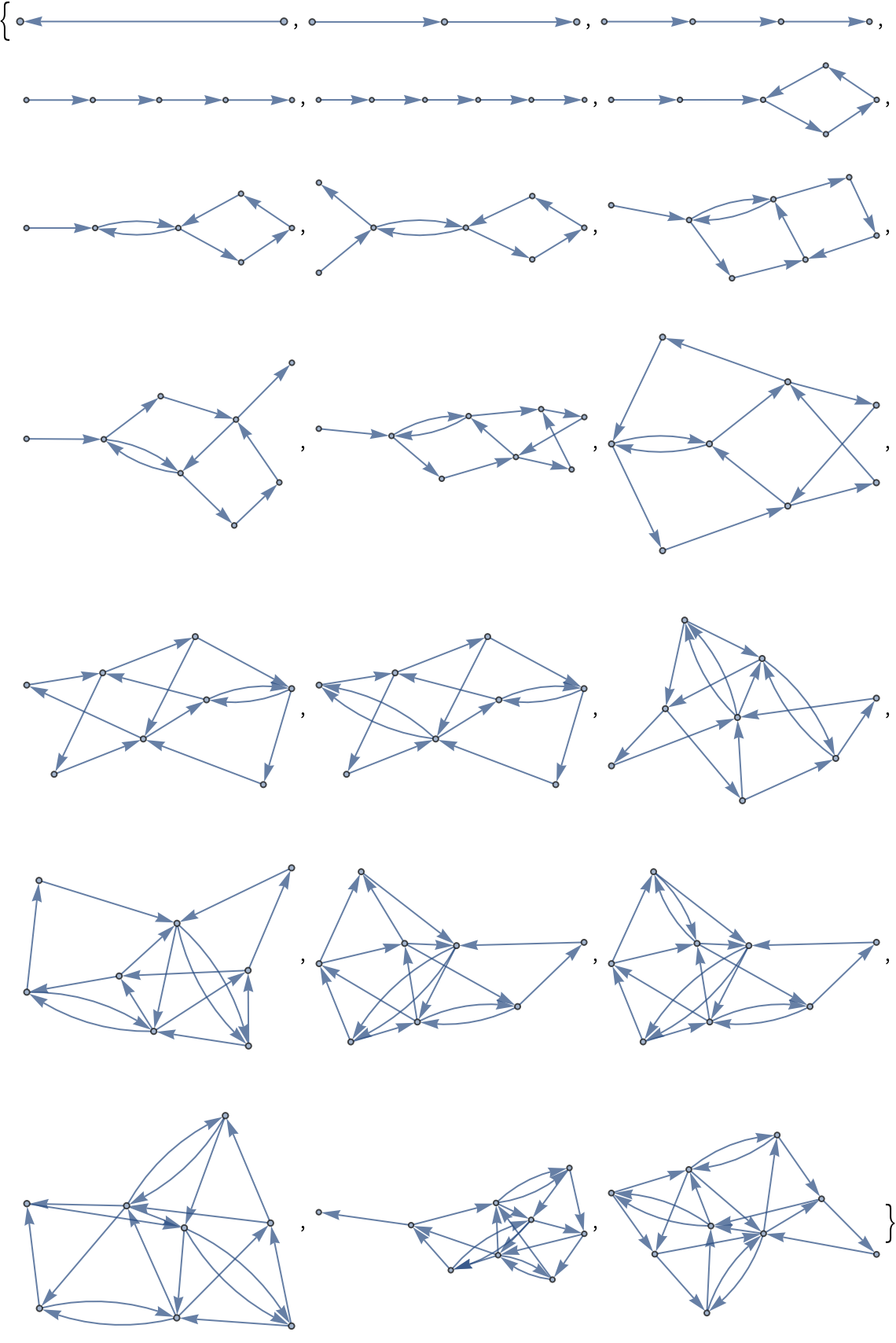Peter Burbery

## Version History

• 1.0.0 – 01 August 2022